# cwtLayer

Continuous wavelet transform (CWT) layer

Since R2022b

## Description

A CWT layer computes the CWT of the input. Use of this layer requires Deep Learning Toolbox™.

## Creation

### Syntax

``layer = cwtLayer``
``layer = cwtLayer(Name=Value)``

### Description

````layer = cwtLayer` creates a CWT layer for a signal of length 1024 samples. The layer uses 10 wavelet filters per octave and periodic boundary conditions. By default, the layer uses the analytic Morse (3,60) wavelet.The input to `cwtLayer` must be a `dlarray` (Deep Learning Toolbox) object in `"CBT"` format. The size along the time dimension of the tensor input is padded to equal the value of SignalLength. By default, `cwtLayer` formats the output as `"SCBT"`. For more information, see Layer Output Format. Note`cwtLayer` initializes the weights internally to be the wavelet filters used in the CWT. It is not recommended to initialize the weights directly. ```

example

````layer = cwtLayer(Name=Value)` creates a CWT layer with properties specified by one or more name-value arguments. For example, ```layer = cwtLayer(Wavelet="amor")``` creates a layer that uses the analytic Morlet wavelet in the CWT. You can specify multiple name-value arguments.```

## Properties

expand all

### CWT

Signal length in samples, specified as a positive integer greater than or equal to 4. All sequence inputs to `cwtLayer` are padded to have size `SignalLength` along the time dimension.

Data Types: `single` | `double`

Analysis wavelet used in the CWT, specified as `"Morse"`, `"amor"`, or `"bump"`, representing the analytic Morse, Morlet (Gabor), and bump wavelet, respectively. The default wavelet is the analytic Morse (3,60) wavelet.

Weight threshold, specified as a positive real scalar. `cwtLayer` uses the threshold value to determine the significant values for each of the CWT filters in the wavelet filter bank prior to any weight modification through learning. It sets values below the specified threshold to zero and excludes them from learning. The CWT filters are normalized so that the peak value is 2 for each filter.

• Smaller values of `Threshold` result in more values being retained from the CWT filters and therefore less weight reduction.

• Larger values of `Threshold` result in more weight reduction and more divergence between the deep learning CWT and transforms computed with the full filter bank.

• A threshold less than `realmin`, the smallest positive normalized floating-point number in double precision, is clipped to `realmin` for computing significant filter values.

Setting `Threshold` to a value which results in no values being retained for any individual filter results in an error.

Data Types: `single` | `double`

Include lowpass filter, specified as a numeric or logical `1` (`true`) or `0` (`false`). Specify `true` to include the lowpass (scaling) filter in the CWT.

Data Types: `logical`

Number of voices per octave in the CWT, specified as an integer between 1 and 48. The CWT scales are discretized using the specified number of voices per octave. The energy spread of the wavelet in frequency and time automatically determines the minimum and maximum scales.

You can use `cwtfreqbounds` to determine the frequency limits of the wavelet filter bank. The frequency limits depend on parameters such as the energy spread of the wavelet, number of voices per octave, and signal length.

Data Types: `single` | `double`

Frequency limits for the CWT, specified as a two-element vector with positive strictly increasing entries. The frequency limits are interpreted as normalized frequencies, cycles/sample.

• The first element specifies the lowest peak passband frequency and must be greater than or equal to the product of the wavelet peak frequency in normalized frequency and two time standard deviations divided by SignalLength.

• The second element specifies the highest peak passband frequency and must be less than or equal to the Nyquist frequency.

The base-2 logarithm of the ratio of the upper frequency limit `freqMax` to the lower frequency limit `freqMin` must be greater than or equal to 1/VoicesPerOctave:

```log2(freqMax/freqMin) ≥ 1/VoicesPerOctave```.

To obtain normalized frequencies, divide your desired frequency limits in hertz by the sample rate in hertz. For example, if the sample rate is 1000 Hz and your desired frequency limits are [100,400] Hz, divide each element by 1000 to obtain the normalized frequencies: [100/1000,400/1000].

Frequency limits apply only to wavelet filters. If you additionally specify `IncludeLowpass` as `true`, `cwtLayer` also includes the lowpass (scaling) filter.

Note

If you use Deep Network Designer (Deep Learning Toolbox) to create or edit a deep learning network, and change the `Wavelet` property of a cwtLayer, the app does not change the frequency limits of the layer. If you want the app to provide the default frequency limits appropriate for the new wavelet, you must take additional steps. For more information, see Reset Frequency Limits to Default Values in Deep Network Designer.

Data Types: `single` | `double`

Time-bandwidth product for the Morse wavelet, specified as a positive scalar greater than or equal to 3 and less than or equal to 120. The symmetry (gamma) of the Morse wavelet is fixed at 3. This property is only valid when `Wavelet` is `"Morse"`. The time-bandwidth product is ignored for the `"amor"` and `"bump"` wavelets. For Morse wavelets, the larger the time-bandwidth product, the more spread out the wavelet is in time and narrower the wavelet is in frequency.

In the notation of Morse Wavelets, `TimeBandwidth` is P2.

Data Types: `single` | `double`

Layer transform mode, specified as one of these:

• `"mag"` — CWT magnitude

• `"squaremag"` — CWT squared magnitude

• `"realimag"` — CWT real and imaginary parts concatenated along the channel dimension

### Layer

Multiplier for weight learning rate, specified as a nonnegative scalar. The weights are the reduced CWT filter values represented as a 1-by-1-by-Nr tensor. See `cwtfilters2array` for details. By default, the weights do not update with training.

Data Types: `single` | `double`

Layer name, specified as a character vector or a string scalar. For `Layer` array input, the `trainnet` (Deep Learning Toolbox), `trainNetwork` (Deep Learning Toolbox), `assembleNetwork` (Deep Learning Toolbox), `layerGraph` (Deep Learning Toolbox), and `dlnetwork` (Deep Learning Toolbox) functions automatically assign names to layers with the name `""`.

The `cwtLayer` object stores this property as a character vector.

Data Types: `char` | `string`

Number of inputs to the layer, returned as `1`. This layer accepts a single input only.

Data Types: `double`

Input names, returned as `{'in'}`. This layer accepts a single input only.

Data Types: `cell`

Number of outputs from the layer, returned as `1`. This layer has a single output only.

Data Types: `double`

Output names, returned as `{'out'}`. This layer has a single output only.

Data Types: `cell`

## Object Functions

 `filterbank` Full-weight CWT filter bank for deep learning

## Examples

collapse all

Create a CWT layer for a signal of length 2000 samples. Set the learning rate factor to 1.

`cLayer = cwtLayer(SignalLength=2000,WeightLearnRateFactor=1);`

Create a three-layer `dlnetwork` containing a sequence input layer, the CWT layer you just made, and a 2-D max pooling layer.

```sqLayer = sequenceInputLayer(1,Name="input",MinLength=2000); mpLayer = maxPooling2dLayer([2 25],Stride=[2 12]); layers = [sqLayer cLayer mpLayer]; dlnet = dlnetwork(layers);```

Run a batch of 10 random single-channel signals through the `dlnetwork`.

```dataout = forward(dlnet, ... dlarray(randn(1,10,2000,"single"),"CBT")); size(dataout)```
```ans = 1×4 40 1 10 165 ```
`dims(dataout)`
```ans = 'SCBT' ```

Load the Espiga3 EEG dataset. The data consists of 23 channels of EEG sampled at 200 Hz. There are 995 samples in each channel. Save the multisignal as a `dlarray`, specifying the dimensions in order. `dlarray` permutes the array dimensions to the `"CBT"` shape expected by a deep learning network.

```load Espiga3 [N,nch] = size(Espiga3); x = dlarray(Espiga3,"TCB"); whos Espiga3 x```
``` Name Size Bytes Class Attributes Espiga3 995x23 183080 double x 23x1x995 183110 dlarray ```

Create a CWT filter bank that is appropriate for the channels in the dataset. Use the default analytic Morse (3,60) wavelet. Specify periodic boundary conditions. Use the filter bank to obtain the CWT of one of the channels. The CWT is a 2-D matrix. The row dimension corresponds to scale, or frequency, and the column dimension corresponds to time.

```fb = cwtfilterbank(SignalLength=N,Boundary="periodic"); colInd = 11; cfs = wt(fb,Espiga3(:,colInd));```

Layer Transform Mode — `"mag"`

Create a CWT layer that can be used with the EEG data. By default, the layer outputs the absolute value of the CWT, or scalogram, of each channel.

`clayer = cwtLayer(SignalLength=N);`

Create a two-layer `dlnetwork` object containing a sequence input layer and the CWT layer you just created. Treat each channel as a feature. Specify the signal length as the minimum sequence length for the input layer.

```slayer = sequenceInputLayer(nch,MinLength=N); layers = [slayer clayer]; dlnet = dlnetwork(layers);```

Run the EEG data through the `forward` method of the network.

`dataout = forward(dlnet,x);`

By default, the output of `cwtLayer` is a `dlarray` object in `"SCBT"` format. The spatial dimension corresponds to frequency. Convert the network output to a numeric array. Permute the dimensions of the network output to correspond with `"STCB"` format. The result is a 3-D numeric array because there is only one batch.

```q = extractdata(dataout); q = permute(q,[1 4 2 3]); whos q cfs```
``` Name Size Bytes Class Attributes cfs 71x995 1130320 double complex q 71x995x23 6499340 single ```

Extract from the output the result that corresponds to the channel you chose. Compare with the absolute value of the CWT you obtained previously.

```r = q(:,:,colInd); d = max(abs(r(:)-abs(cfs(:)))); str = sprintf("Difference: %g",d); fprintf("%s\n",str)```
```Difference: 8.86966e-06 ```
```subplot(2,1,1) imagesc(r) title("Layer Output") subplot(2,1,2) imagesc(abs(cfs)) title("Filter Bank Output")```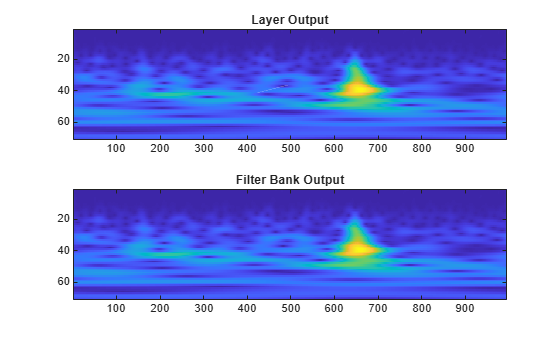Layer Transform Mode — `"realimag"`

Create a CWT layer that can be used with the data. Specify the layer outputs to be the real and imaginary parts of the CWT. Create a two-layer `dlnetwork` object containing a sequence input layer and the CWT layer. Run the EEG data through the `forward` method of the network.

```clayer2 = cwtLayer(SignalLength=N,TransformMode="realimag"); layers2 = [slayer clayer2]; dlnet2 = dlnetwork(layers2); dataout2 = forward(dlnet2,x);```

Convert the network output to a numeric array. Permute the dimensions of the network output to correspond with `"STCB"` format. Because the output are the real and imaginary parts of the CWT, the size of the channel dimension is twice the number of input channels.

```q2 = extractdata(dataout2); q2 = permute(q2,[1 4 2 3]); whos q2```
``` Name Size Bytes Class Attributes q2 71x995x46 12998680 single ```

Choose a channel. Use the CWT filter bank to obtain the CWT of that channel. Compare the real and imaginary parts of the CWT with the corresponding results from the network output.

```colInd = 23; cfs = wt(fb,Espiga3(:,colInd)); r = q2(:,:,[colInd nch+colInd]); a1 = real(cfs); a2 = r(:,:,1); str1 = sprintf("Difference (real part): %g",max(abs(a1(:)-a2(:)))); a1 = imag(cfs); a2 = r(:,:,2); str2 = sprintf("Difference (imag part): %g",max(abs(a1(:)-a2(:)))); fprintf("%s\n%s\n",str1,str2)```
```Difference (real part): 7.102e-05 Difference (imag part): 6.94453e-05 ```
```figure subplot(2,2,1) imagesc(real(cfs)) title("Filter Bank — Real") subplot(2,2,2) imagesc(r(:,:,1)) title("Layer — Real") subplot(2,2,3) imagesc(imag(cfs)) title("Filter Bank — Imag") subplot(2,2,4) imagesc(r(:,:,2)) title("Layer — Imag")```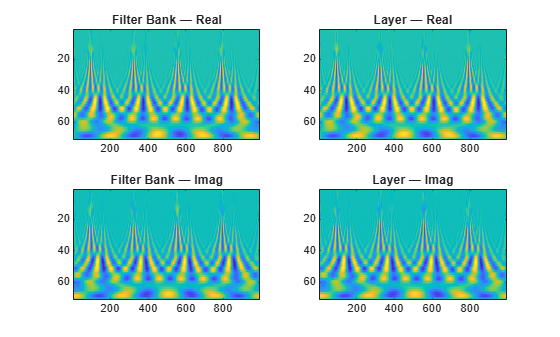Create a CWT layer using default values. By default, the CWT layer is for a signal length of 1024 samples. The layer uses the analytic Morse (3,60) wavelet in the CWT with 10 voices per octave and periodic boundary conditions. Inspect the default frequency limits of the layer. The frequency limits, which are in units of cycles/sample, are based on the energy spread of the wavelet, the signal length, and the voices per octave.

```clayer = cwtLayer; clayer.FrequencyLimits```
```ans = 1×2 0.0032 0.4341 ```

Use the `filterbank` method of the layer to obtain the full-weight CWT filter bank for the layer. Each row contains the values of a filter. The filters are ordered from high center frequency to low.

```psif = filterbank(clayer); whos psif```
``` Name Size Bytes Class Attributes psif 71x1024 290816 single ```

You can determine the center frequencies by creating a `cwtfilterbank` object, specifying the same wavelet parameters as used in the CWT layer, and then use the `centerFrequencies` object function.

Create a `cwtfilterbank`. The default wavelet parameters are identical to those in the CWT layer. Obtain the center frequencies. The frequencies are ordered from high to low. Plot the center frequencies.

```fb = cwtfilterbank; cf = centerFrequencies(fb); subplot(2,1,1) plot(cf) ylabel("Cycles/Sample") title("Wavelet Center Frequencies") grid on subplot(2,1,2) semilogy(cf) grid on ylabel("Cycles/Sample") title("Semi-Log Scale")```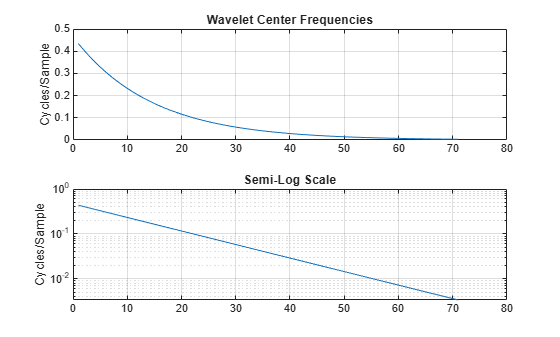The plots show that the wavelet center frequencies are not linearly spaced as is commonly the case with other filter banks. Specifically, the center frequencies are exponentially decreasing. In continuous wavelet analysis, the center frequencies of the wavelet filters are logarithmically spaced. The most common spacing is the base 2^(1/NV), where NV is the number of voices per octave, raised to integer powers. In other words, in a CWT filter bank, it is not possible for consecutive center frequencies ${f}_{1}$ and ${f}_{2}$, where ${f}_{1}<{f}_{2}$, to satisfy ${\mathrm{log}}_{2}\left({f}_{2}/{f}_{1}\right)<1/NV$. Frequency limits you specify in the CWT layer must satisfy ${\mathrm{log}}_{2}\left({f}_{2}/{f}_{1}\right)\ge 1/NV$.

The CWT layer uses 10 voices per octave. Compute the base-2 logarithm of the ratios of consecutive pairs of center frequencies. Confirm the minimum and maximum values of the result are both equal to 1/10.

```cfRatio = log2(cf(1:end-1)./cf(2:end)); [min(cfRatio) max(cfRatio)]```
```ans = 1×2 0.1000 0.1000 ```

Plot the full-weight CWT filter bank and the center frequencies. The center frequencies correspond to the peaks of the frequency response of each wavelet in the filter bank.

```slen = clayer.SignalLength; f = 0:1/slen:1-1/slen; figure plot(f,psif') xlim([0 1/2]) xlabel("Cycles/Sample") ylabel("Magnitude") title(["Full-Weight Filter Bank", "With Center Frequencies"]) hold on plot(cf,2*ones(size(psif,1),1),'bx') hold off```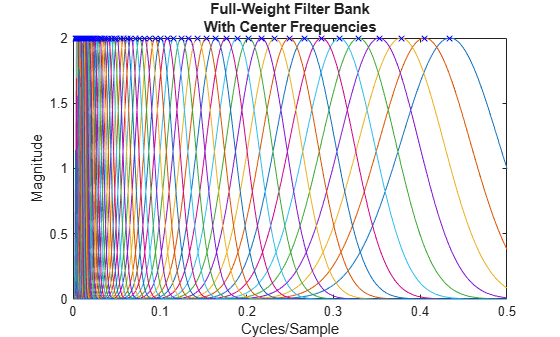If the CWT layer you created does not support your frequency limits, try increasing the number of voices per octave in the layer. For more information, see Practical Introduction to Time-Frequency Analysis Using the Continuous Wavelet Transform and Continuous and Discrete Wavelet Transforms.

This example shows how to reset the frequency limits to default values after changing the wavelet of a cwtLayer in Deep Network Designer.

Suppose you are editing a deep learning network using Deep Network Designer. The network has a cwtLayer that uses the Morse wavelet and has frequency limits set at `[0.1,0.3]`.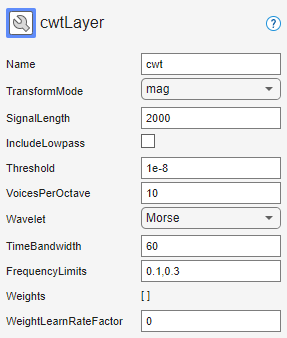Use the drop-down list to change the Wavelet to `amor`. The frequency limits do not change, but the number of weights does change.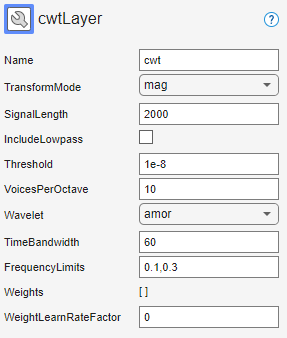To change the frequency limits to default values appropriate for the `amor` wavelet, first do one of the following:

• Delete the FrequencyLimits `0.1,0.3`.

• Set the FrequencyLimits to `[]`.

Then click the mouse outside the FrequencyLimits edit field to move the focus. The app automatically populates FrequencyLimits with default values for the `amor` wavelet. The number of weights also changes to reflect the new limits.

Tip: If you change the frequency limits, and later want to restore the default values, follow the same steps.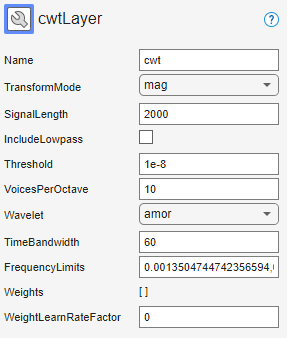expand all

## Version History

Introduced in R2022b